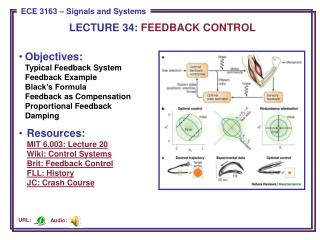DownloadDownload PresentationLECTURE 34: FEEDBACK CONTROL

# LECTURE 34: FEEDBACK CONTROL

Download Presentation## LECTURE 34: FEEDBACK CONTROL

- - - - - - - - - - - - - - - - - - - - - - - - - - - E N D - - - - - - - - - - - - - - - - - - - - - - - - - - -
##### Presentation Transcript

1. LECTURE 34: FEEDBACK CONTROL • Objectives:Typical Feedback SystemFeedback ExampleBlack’s FormulaFeedback as CompensationProportional FeedbackDamping • Resources:MIT 6.003: Lecture 20Wiki: Control SystemsBrit: Feedback ControlFLL: HistoryJC: Crash Course Audio: URL:

2. A Typical Feedback System Feed Forward Feedback • Why use feedback? • Reducing Nonlinearities • Reducing Sensitivity to Uncertainties and Variability • Stabilizing Unstable Systems • Reducing Effects of Disturbances • Tracking • Shaping System Response Characteristics (bandwidth/speed)

3. Motivating Example • Open loop system: aim and shoot. • What happens if you miss? • Can you automate the correctionprocess? • Closed-loop system: automatically adjusts until the proper coordinates are achieved. • Issues: speed of adjustment, inertia, momentum, stability, …

4. System Function For A Closed-Loop System • The transfer function of thissystem can be derived usingprinciples we learned inChapter 6: • Black’s Formula: Closed-loop transfer function is given by: • Forward Gain: total gain of the forward path from the inputto the output, where the gain of a summer is 1. • Loop Gain: total gain along the closed loop shared by all systems. Loop

5. The Use Of Feedback As Compensation • Assume the open loopgain is very large(e.g., op amp):  Independent of P(s) • The closed-loop gain depends only on the passive components (R1 and R2) and is independent of the open-loop gain of the op amp.

6. Stabilization of an Unstable System • If P(s) is unstable, can westabilize the system byinserting controllers? • Design C(s) and G(s) so thatthe poles of Q(s) are in the LHP: • Example: Proportional Feedback (C(s) = K) • The overall system gain is: • The transfer function is stable for K > 2. • Hence, we can adjust K until the system is stable.

7. Second-Order Unstable System • Try proportional feedback: • One of the poles is at • Unstable for all values of K. • Try damping, a term proportional to : • This system is stable as long as: • K2 > 0: sufficient damping force • K1 > 4: sufficient gain • Using damping and feedback, we have stabilized a second-order unstable system.

8. Summary • Introduced the concept of system control using feedback. • Introduced a method of calculating the system gain known as Black’s formula. • Demonstrated how we can stabilize first-order systems using simple proportional feedback, and second-order systems using damping (derivative proportional feedback). • Why did we not simply cancel the poles? • In real systems we never know the exact locations of the poles. Slight errors in predicting these values can be fatal. • Disturbances between the two systems can cause instability. • There are many ways we can use feedback to control systems including feedback that adapts over time to changes in the system or environment.